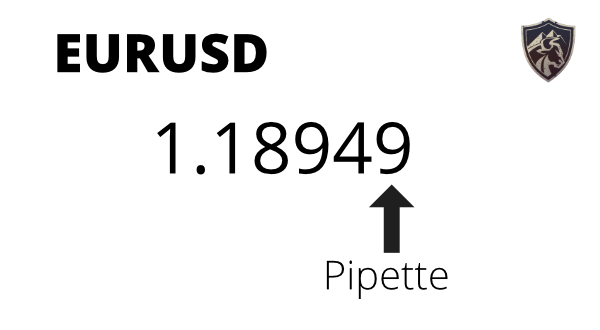# What is a Pip in Forex Trading?

12
0Profit and loss in Forex trading is calculated in pips, which can be a little confusing to beginners. So in this post, I’ll show you how pips work and how to calculate profit and loss in pips.

A pip is the smallest price move in the Forex market. It is short for “price interest point.” In currency pairs that don’t have the Japanese Yen in it, 1 pip is a 0.0001 change in the price of the currency. When the Japanese Yen is one of the currencies in a pair, 1 pip is a 0.01 change in the price. Smaller price changes are called pipettes.

This video will give you more examples on how calculate your profit and loss in pips.

?

## How to Calculate Profit and Loss in Pips

Calculating your profit and loss on a trade starts with calculating the number of pips that you’ve made or lost. Here’s how you do it:

### Step 1: Subtract the Open From the Close

To illustrate how this works, I’m going to use a theoretical example trade:

• Long
• EURUSD
• Open price: 1.18443
• Close price: 1.18555

The price you start with will depend on if you went long or short.

Then subtract the other price to get your profit and loss in pips.

Then I’ll subtract the open price: 1.18555 – 1.18443 = 0.00112.

Since that’s a positive number, that means the trade was profitable. This makes sense, since the close was higher than the open, on a long trade. A negative number means that you have a losing trade.

I know this is probably obvious to you, but there will be people who ask why I start with the close for a long and the open for a short. So I’m including that information to make this a complete tutorial for beginners.

### Step 2: Multiply by a Constant to Get Number of Pips

Alright, since this is a non-JPY pair, we multiply the number by 10,000 to get the number of pips: 0.00112 X 10,000 = 11.2.

So on this trade, there was a profit of 11.2 pips.

When dealing with JPY pairs, you would multiply by 100 in the last step to get the number of pips profit or loss.

That’s it!

## What’s a Pipette?

A pipette is a fraction of a pip, 1/10 of a pip, to be exact.

Many brokers quote prices in pipettes to help give their customers tighter spreads.

For example, if a broker used only whole pips, the spread on the EURUSD could only be 1 or 2. But with pipettes, they can provide a spread of 1.5 pips, potentially saving you 0.5 pips on every trade.Another way to think of a pipette is like a fraction of a penny in the US stock market. The smallest price move in a stock is 1 penny.

However, in 2005 the SEC created Rule 612. This rule states that stocks worth less than \$1 have to be quoted in minimum increments of \$0.0001.

So this fraction of a penny is similar to a pipette, which is a fraction of a pip.

## How Many Pips a Day is Good?

This is a common question among new traders and I understand where they are coming from. However, setting a pip goal is not useful in real-world trading.

Here’s why…

Dollar amounts don’t matter, pips don’t matter and number of trades don’t matter. All that matters is if you’re managing your risk correctly by taking the right trade size for your account.

For example, let’s say that you made 50 pips on a trade and you have a \$10,000 account.

Well, how much money is that?

What percentage of your account is that?

There’s no connection between those 50 pips and your \$10,000 account. However, when you calculate how much you gained on that trade, then you can start to understand how much of an impact that trade had on your account and if that was a good amount to risk, based on your backtesting.

So in order to find out your percentage gain on your account you would do the following:

(pips profit or loss) X (cost per pip, per lot) X (number of lots) = \$ profit or loss

(50 pips profit) X (\$0.10 per pip, per mini lot) X (10 mini lots) = +\$50 profit

Then divide the profit by the total account balance to get your percentage profit:

\$50 / \$10,000 = 0.5% profit.

Now we can see that 50 pips of profit was actually a very small gain, in relation to the total size of the account. So you might want to risk more on future trades.

But always backtest before making changes to your strategy.

## Conclusion

So that’s how pips work in the Forex market. They are the starting point for calculating your profit and loss on a trade, but they are not an important metric when tracking performance.

I can always spot someone who doesn’t actually trade when they create a trading journal or trading report that tracks pips 🙂